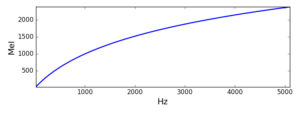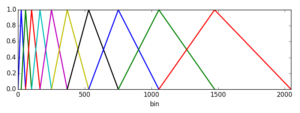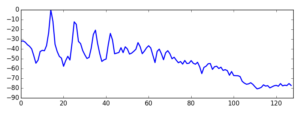## The entrails of Open Sound Control, part one

Many of us are very used to employing the Open Sound Control (OSC) protocol to communicate with synthesisers and other music software. It’s very handy and flexible for a number of applications. In the cross adaptive project, OSC provides the backbone of communications between the various bits of programs and plugins we have been devising.

Generally speaking, we do not need to pay much attention to the implementation details of OSC, even as developers. User-level tasks only require us to decide the names of messages addresses, its types and the source of data we want to send. At Programming level,  it’s not very different: we just employ an OSC implementation from a library (e.g. liblo, PyOSC) to send and receive messages.

It is only when these libraries are not doing the job as well as we’d like that we have to get our hands dirty. That’s what happened in the past weeks at the project. Oeyvind has diagnosed some significant delays and higher than usual cost in OSC message dispatch. This, when we looked, seemed to stem from the underlying implementation we have been using in Csound (liblo, in this case). We tried to get around this by implementing an asynchronous operation, which seemed to improve the latencies but did nothing to help with computational load. So we had to change tack.

OSC messages are transport-agnostic, but in most cases use the User Datagram Protocol transport layer to package and send messages from one machine (or program) to another. So, it appeared to me that we could just simply write our own sender implementation using UDP directly. I got down to programming an OSCsend opcode that would be a drop-in replacement for the original liblo-based one.

OSC messages are quite straightforward in their structure, based on 4- byte blocks of data. They start with an address, which is a null-terminated string like, for instance, “/ foo / bar ”  :

``` '/' 'f' 'o' 'o' '/' 'b' 'a' 'r' '\0' ```

This, we can count, has 9 characters – 9 bytes – and, because of the 4-byte structure, needs to be padded to the next multiple of 4, 12, by inserting some more null characters (zeros). If we don’t do that, an OSC receiver would probably barf at it.

Next, we have the data types, e.g. ‘i’, ‘f’, ‘s’ or ‘b’ (the basic types). The first two are numeric, 4-byte integers and floats, respectively. These are to be encoded as big-endian numbers, so we will need to byteswap in little-endian platforms before the data is written to the message. The data types are encoded as a string with a starting comma (‘,’) character, and need to conform to 4-byte blocks again. For instance, a message containing a single float would have the following type string:

``` ',' 'f' '\0' ```

or “,f”. This will need another null character to make it a 4-byte block. Following this, the message takes in a big-endian 4-byte floating-point number.  Similar ideas apply to the other numeric type carrying integers.

String types (‘s’) denote a null-terminated string, which as before, needs to conform to a length that is a multiple of 4-bytes. The final type, a blob (‘b’), carries a nondescript sequence of bytes that needs to be decoded at the receiving end into something meaningful. It can be used to hold data arrays of variable lengths, for instance. The structure of the message for this type requires a length (number of bytes in the blob) followed by the byte sequence. The total size needs to be a multiple of 4 bytes, as before. In Csound, blobs are used to carry arrays, audio signals and function table data.

If we follow this recipe, it is pretty straightforward to assemble a message, which will be sent as a UDP packet. Our example above would look like this:

``` '/' 'f' 'o' 'o' '/' 'b' 'a' 'r' '\0' '\0' '\0' '\0' ',' 'f' '\0' '\0' 0x00000001 ```

This is what OSCsend does, as well as its new implementation. With it, we managed to provide a lightweight (low computation cost) and fast OSC message sender. In the followup to this post, we will look at the other end, how to receive arbitrary OSC messages from UDP.

## Adding new array operations to Csound II: the Mel-frequency filterbank

As I have discussed before in my previous post , as part of this project we have been selecting a number of useful operations to implement in Csound, as part of its array opcode collection. We have looked at the components necessary for the implementation of the Mel-frequency cepstrum coefficient (MFCC) analysis and in this post I will discuss the Mel-frequency filterbank as the final missing piece.

The word filterbank might be a little misleading in this context, as we will not necessarily implement a complete filter. We will design a set of weighting curves that will be applied to the power spectrum. From each one of this we will obtain an average value, which will be the output of the MFB at a given centre frequency. So from this perspective, the complete filter is actually made up of the power spectrum analysis and the MFB proper.

So what we need to do is the following:

1. Find L evenly-space centre frequencies in the Mel scale (within a minimum and maximum range).
2. Construct L overlapping triangle-shape curves, centred at each Mel-scale frequency.
3. Apply each one of these curves to the power spectrum and averaging the result. These will be the outputs of the filterbank.

The power spectrum input comes as sequence of equally-spaced bins. So, to achieve the first step, we need to convert to/from the Mel scale, and also to be able to establish which bins will be equivalent to the centre frequencies of each triangular curve. We will show how this is done using the Python language as an example.

The following function converts from a frequency in Hz to a Mel-scale frequency.

```import pylab as pl
def f2mel(f):
return 1125.*pl.log(1.+f/700.)
```With this function, we can convert our start and end Mel values and linearly space the L filter centre frequencies. From these L Mel values, we can get the power spectrum bins using

```def mel2bin(m,N,sr):
f = 700.*(pl.exp(m/1125.) - 1.)
return  int(f/(sr/(2*N)))
```

where m is the Mel frequency, N is the DFT size used and sr is the sampling rate. A list of bin numbers can be created, associating each L Mel centre frequency with a bin number

Step 2 is effectively based on creating ramps that will connect each bin in the list above. The following figure demonstrates the idea for L=10, and N=4096 (2048 bins)Each triangle starts at a Mel frequency in the list, rises to the next, and decays to the following one (frequencies are quantised to bin centres). To obtain the output for each filter we weigh the bin values (spectral powers) by these curves and then output the average value for each band.

The Python code for the MFB operation is shown below:

```def MFB(input,L,min,max,sr):
"""
From a power spectrum in input, creates an array
consisting of L values containing
its MFB, from a min to a max frequency sampled
at sr Hz.
"""
N = len(input)
start = f2mel(min)
end = f2mel(max)
incr = (end-start)/(L+1)
bins = pl.zeros(L+2)
for i in range(0,L+2):
bins[i] = mel2bin(start,N-1,sr)
start += incr
output = pl.zeros(L)
i = 0
for i in range(0,L):
sum = 0.0
start = bins[i]
mid = bins[i+1]
end = bins[i+2]
incr =  1.0/(mid - start)
decr =  1.0/(end - mid)
g = 0.0
for bin in input[start:mid]:
sum += bin*g
g += incr
g = 1.0
for bin in input[mid:end]:
sum += bin*g
g -= decr
output[i] = sum/(end - start)
return output
```

We can demonstrate the use of the MFB  by plotting the output of a N=4096, L=128 full-spectrum magnitude analysis of a flute tone.We can see how the MFB identifies clearly the signal harmonics. Of course, the original application we had in mind (MFCCs) is significantly different from this one, but this example shows what kinds of outputs we should expect from the MFB.